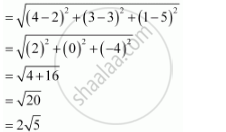CBSE (Arts) Class 11CBSE
Share

# Find the Distance Between the Pairs of Points (2, 3, 5) and (4, 3, 1) - CBSE (Arts) Class 11 - Mathematics

ConceptThree - Dimensional Geometry Distance Between Two Points

#### Question

Find the distance between the pairs of points

(2, 3, 5) and (4, 3, 1)

#### Solution

The distance between points P(x1y1z1) and P(x2y2z2) is given by

PQ = sqrt((x_2 -x_1)^2 + (y_2-y^1)^2 + (z_2 - z_1)^2)

Distance between points (2, 3, 5) and (4, 3, 1)Is there an error in this question or solution?

#### APPEARS IN

NCERT Solution for Mathematics Textbook for Class 11 (2018 to Current)
Chapter 12: Introduction to Three Dimensional Geometry
Q: 1.1 | Page no. 273

#### Video TutorialsVIEW ALL 

Solution Find the Distance Between the Pairs of Points (2, 3, 5) and (4, 3, 1) Concept: Three - Dimensional Geometry - Distance Between Two Points.
S